Downhill Race

Slow Acceleration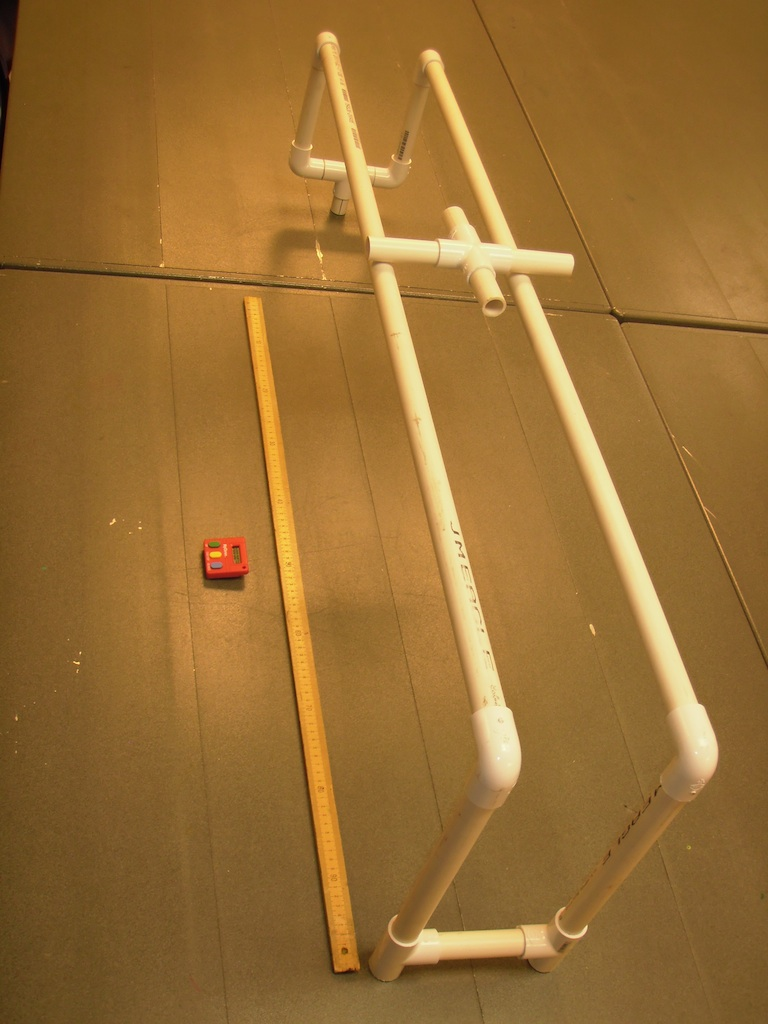The PVC downhill race

Introduction

Slow acceleration can be studied by rolling an object down a gently inclined plane.

Material

PVC cutting tool
15 feet ( 5 meters) of Schedule 40 3/4 inch PVC tubing (You can build a longer ramp by using more PVC)
6   90 degree angles 3/4 PVC slip joints
3   T junctions 3/4 PVC slip joints
1     Cross  3/4 PVC
stopwatch
tape measure

Assembly

Ramp

Cut two equal length pieces of PVC at least 4 feet long.
Attach a downward pointing 90 degree angle to both ends of each rail.

Low end

Connect two vertical tubes to the 90 degree couplings at the ends of the ramps, perhaps 8 inches (20 cm) long.
Connect the bottoms of each vertical to two 90 degree corners and connect these to a T connector.
Insert a short PVC pipe into the bottom of the T connector to give the low end the height you desire.
Mine was 12 inches ( 30 cm).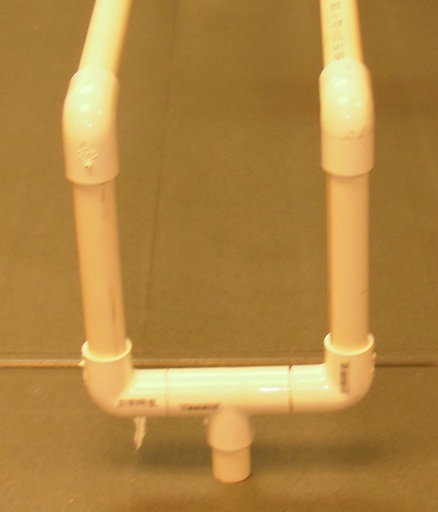High end

Cut two vertical risers of equal length and insert them into the 90 degree angles from the ramps connect them to T connectors at the bottom.
Connect the T's with a horizontal pipe. Choose the length of the pipe to keep the two rails parallel. See detail below.
I adjusted the high end to be 15 inches (38 cm) above the table. The high end should be at least a couple of inches (5cm) higher than the low end.
The higher the high end above the low end, the faster the acceleration.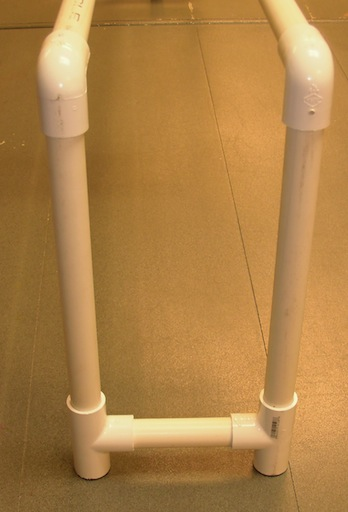Roller

Insert two longer pipes into the cross connector. Mine were 3.5 inches long.
Perpendicular to the axis insert two identical length pipes. (If they are not the same length the roller might refuse to roll or even start to roll uphill.)
Short pipes or no pipes at all create a more rapid acceleration.
Long pipes slow the acceleration.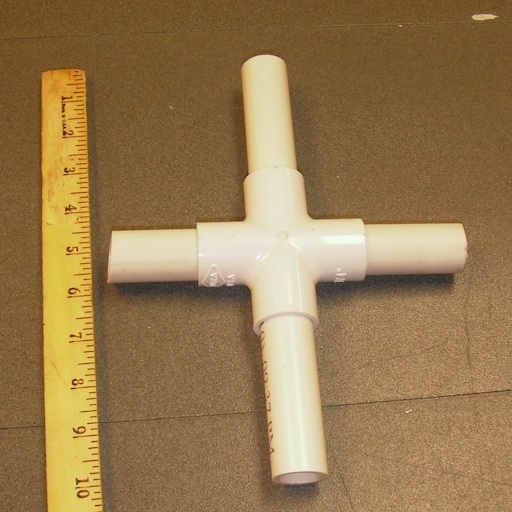The axles should be long enough that if the roller drifts to the side far enough that the edge of the cross touches a rail
the axle will not fall off the rails, as shown below.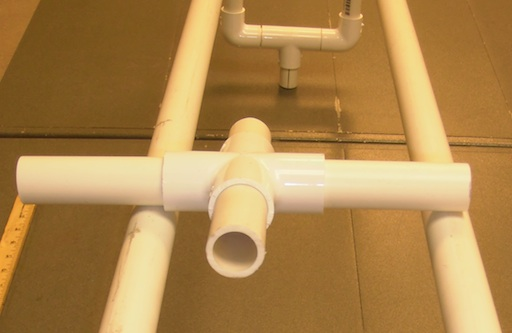To Do and Notice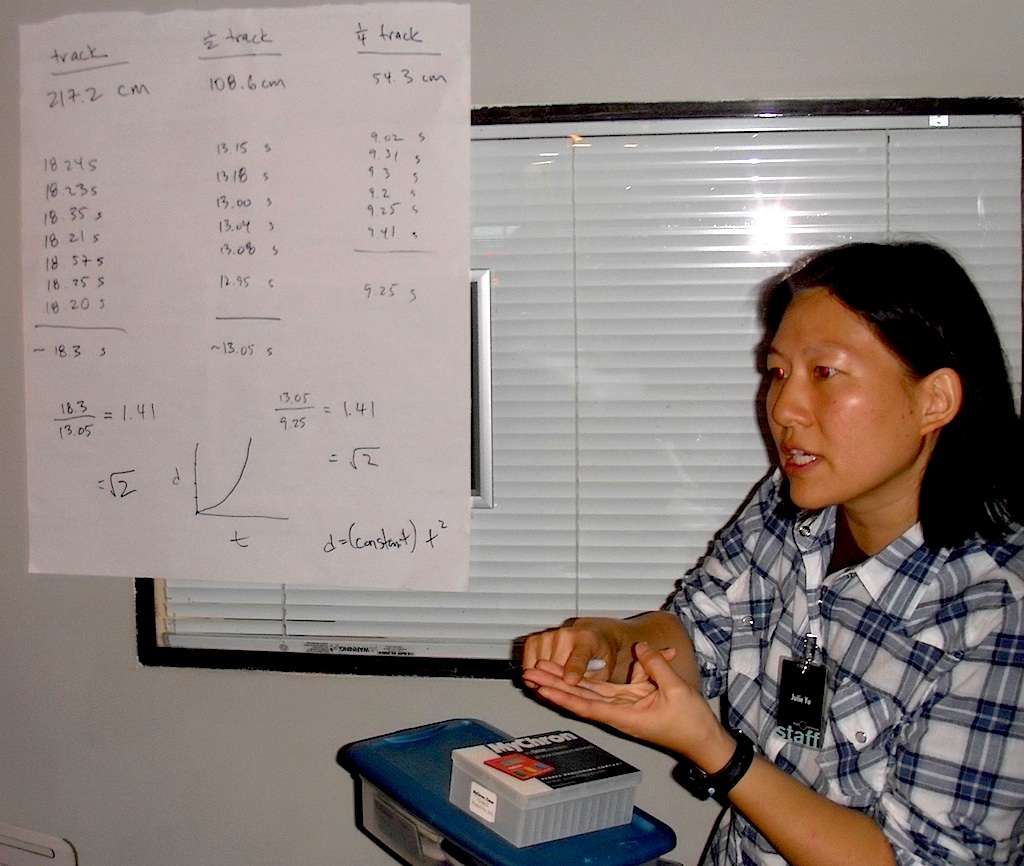Julie Yu has teachers do the measurements and the math at downhill racer.(Click to enlarge)

Measure the length of the ramp. L1, (It's PVC you can adjust the length to be anything you want.) Let's say 48 inches (122 cm).
Start the roller at the high end of the ramp and start a stopwatch.
Measure how long it takes the roller to reach the bottom of the ramp.
Do this a few times and take the average time. Perhaps 9.0 seconds. This is the average time.
(To computer the average time for 3 measurements , add the three measurements and divide the sum by 3.)

Now find the center of the ramp.
In my ramp it is 24 inches (61 cm) from the start, L2.
Start the roller from rest and measure the time to roll  to the center, 1/2 the length of the ramp.
Do it several times and average the result. My result was 6.5 s.

Now measure the average time to start from rest and roll the first 1/4 of the ramp, 12 inches (30 cm), L4.
Mine was 4.5 seconds

Take the ratio of the time for the entire ramp, T1, to the time to roll 1/2 the ramp T2.
T1/T2 = 9.0/6.5 = 1.4

Now take the ratio for the time to roll 1/2 the ramp, T2, to the time for a quarter of the ramp,T4.
T2/T4 = 6.5/4.5 = 1.4

Notice the ratios remain the same.
Notice also that 1.4 is close to the square root of 2.

The ratios of the times is the square root of the ratios of the distances.

L1/L2 = 2

T1/T2 = Square root of 2 = 1.4

so L1/L2 = (T1/T2)^2

or L = k T^2  length traveled from rest is proportional to the time squared.

This is the fundamental rule for constant acceleration.

What's Going On?

Objects accelerating down an inclined plane accelerate slower than the acceleration of gravity for a dropped object.
Galileo knew this and used to to find the mathematics of objects undergoing constant acceleration.

the basic equation relating distance traveled from rest d to the constant acceleration a and the time t is

d = 1/2 a t^2

solving for the acceleration in my case we find a = 2d/t^2 = 2* 2.44 m/(9.0 s)^2 = 0.06 m/s^2.

This is 0.006 g or less than 1% of the acceleration of gravity in freefall.

We have achieved a very low acceleration indeed.

So What?

The same rule is followed for objects in freefall, when friction can be ignored with a much larger acceleration of 9.8 m/s^2.

Going Further

Start the object from rest and have the teachers mark the position at constant time intervals. In my case after every 2 seconds.

So, I got marks at

 2s 0.12 m 1 4s 0.48 m 4 6s 1.1   m 9.2 8s 1.9   m 16

The last column shows the distances traveled in units of the first measurement, 0.12m.

Notice that the distance traveled is proportional to the square of the number of intervals of time traveled The sequence 1,4,9,16 is the sequence of the squares of the integers.

Galileo measured this and saw that the distance traveled under constant acceleration was proportional to the square of the time.

 Scientific Explorations with Paul Doherty © 2011 19 Oct 2011